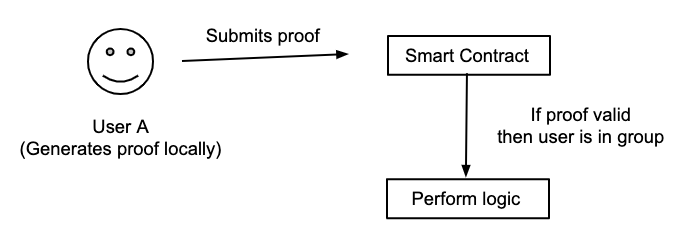## 概述<center>图 1: Zk-dApp身份验证-用户流程图</center>

1. 编写零知识电路
2. 生成用于验证零知识电路的`Solidity`代码库
3. 编写智能合约逻辑，并集成步骤 2生成的 `Solidity` 代码库
4. 部署合约.
5. 本地生成证明，并在链上进行验证。

### 开发环境搭建及工具

• 使用`JavaScript/TypeScript` 开发 DApp，本身有丰富的生态以及对以太坊也有非常好的支持
• 使用`Solidity` 开发智能合约，足够成熟、社区很好
• 使用`Truffle` 部署合约
• 使用`Circom` 开发零知识证明电路

## 零知识证明电路

``````// 请注意，私钥是标量值(int)
// 而公钥是空间中的一个点(Tuple[int, int])
const zk_identity = (private_key, public_keys) => {
// derive_public_from_private 是一个用来返回私钥对应公钥的函数
derived_public_key = derive_public_from_private(private_key)
for (let pk in public_keys):
if derived_public_key === pk:
return true
return false
}``````

``````npm install -g circom  snarkjs
npm install circomlib  websnark

mkdir contracts
mkdir circuits
mkdir -p build/circuits

touch circuits/circuit.circom``````

``````include "../node_modules/circomlib/circuits/bitify.circom";
include "../node_modules/circomlib/circuits/escalarmulfix.circom";
include "../node_modules/circomlib/circuits/comparators.circom";

template PublicKey() {
// 注意：需要对私钥进行哈希，然后对其修剪以确保其与baby JubJub曲线兼容
signal private input in;
signal output out;

component privBits = Num2Bits(253);
privBits.in &lt;== in;

var BASE8 = [
5299619240641551281634865583518297030282874472190772894086521144482721001553,
16950150798460657717958625567821834550301663161624707787222815936182638968203
];

component mulFix = EscalarMulFix(253, BASE8);
for (var i = 0; i &lt; 253; i++) {
mulFix.e[i] &lt;== privBits.out[i];
}

out &lt;== mulFix.out;
out &lt;== mulFix.out;
}``````

<center> 项目根目录/circuits/circuit.circom</center>

PublicKey 电路模板的作用是在baby JubJub 曲线上从提供的私钥（`in`）导出公钥（`out`）（即上述伪代码中的 `derive_public_from_private` 函数）。

``````include ...

template PublicKey() {
...
}

template ZkIdentity(groupSize) {
// 公钥在智能合约中
// 注意: 假设这些公钥都是唯一的
signal input publicKeys[groupSize];

// 证明者的私钥（这是一个私有的输入信号）
signal private input privateKey;

// 从证明者的私钥推导出的公钥
component publicKey = PublicKey();
publicKey.in &lt;== privateKey;

// 确保推导的公钥需要与智能合约中的至少一个公钥匹配以验证其身份
var sum = 0;

// 创建一个组件以检查两个值是否相等
component equals[groupSize];
for (var i = 0; i &lt; groupSize; i++) {

// 我们不使用 === 因为如果不为true, 他会立即失败
equals[i] = IsEqual();
equals[i] = IsEqual();

equals[i].in &lt;== publicKeys[i];
equals[i].in &lt;== publicKey.out;

equals[i].in &lt;== publicKeys[i];
equals[i].in &lt;== publicKey.out;

sum += equals[i].out;
sum += equals[i].out;
}

// 如果相等，equals[i][j].out 会返回 1，如果不等返回 0
// 因此, 如果派生的公钥（空间中的某个点）与智能合约中列出的公钥匹配，则所有equals[i][j].out的总和应等于2
sum === 2;
}

// Main 入口
component main = ZkIdentity(2);``````

<center> 项目根目录/circuits/circuit.circom</center>

``````\$ circom circuits/circuit.circom --r1cs --wasm --sym

## snarkjs setup might take a few seconds
\$snarkjs setup --protocol groth -r circuit.r1cs --pk build/circuits/provingKey.json --vk build/circuits/verifyingKey.json

## 生成 solidity 代码用来验证证明
\$snarkjs generateverifier --pk build/circuits/provingKey.json --vk build/circuits/verifyingKey.json -v contracts/Verifier.sol

## 应该可以看到在contracts目录下生成了一个合约文件 "Verifier.sol"
## \$ ls contracts
## Migrations.sol Verifier.sol``````

## 智能合约验证

``````...

function verifyProof(
uint memory a,
uint memory b,
uint memory c,
uint memory input
) public view returns (bool r) {
Proof memory proof;
proof.A = Pairing.G1Point(a, a);
proof.B = Pairing.G2Point([b, b], [b, b]);
proof.C = Pairing.G1Point(c, c);
uint[] memory inputValues = new uint[](input.length);
for(uint i = 0; i &lt; input.length; i++){
inputValues[i] = input[i];
}
if (verify(inputValues, proof) == 0) {
return true;
} else {
return false;
}
}

...``````

<center> 项目根目录/contracts/Verifier.sol</center>

``````pragma solidity 0.5.11;

import "./Verifier.sol";

contract ZkIdentity is Verifier {
uint256 public publicKeys;

constructor() public {
owner = msg.sender;
publicKeys = [
[
11588997684490517626294634429607198421449322964619894214090255452938985192043,
15263799208273363060537485776371352256460743310329028590780329826273136298011
],
[
3554016859368109379302439886604355056694273932204896584100714954675075151666,
17802713187051641282792755605644920157679664448965917618898436110214540390950
]
];
}

function isInGroup(
uint256 memory a,
uint256 memory b,
uint256 memory c,
uint256 memory input // public inputs
) public view returns (bool) {
if (
input != publicKeys &&
input != publicKeys &&
input != publicKeys &&
input != publicKeys
) {
revert("Supplied public keys do not match contracts");
}

return verifyProof(a, b, c, input);
}
}``````

<center> 项目根目录/contracts/ZkIdentity.sol</center>

## 生成证明并与智能合约交互

``````// 假定这些都已经存在了
const provingKey // provingKey.json
const circuit // 我们编写的零知识证明电路
const zkIdentityContract // Zk-Identity 合约实例
const privateKey  // 与智能合约中的公钥之一相对应的私钥

const publicKeys = [
[
11588997684490517626294634429607198421449322964619894214090255452938985192043n,
15263799208273363060537485776371352256460743310329028590780329826273136298011n
],
[
3554016859368109379302439886604355056694273932204896584100714954675075151666n,
17802713187051641282792755605644920157679664448965917618898436110214540390950n
]
]

const circuitInputs = {
privateKey,
publicKeys
}

const witness = circuit.calculateWitness(circuitInputs)
const proof = groth16GenProof(witness, provingKey)

const isInGroup = zkIdentityContract.isInGroup(
proof.a,
proof.b,
proof.c,
witness.publicSignals
)``````

## 结论

• 发表于 2020-06-10 14:56
• 阅读 ( 1682 )
• 学分 ( 184 )
• 分类：零知识证明